# Derivability Over An Interval JEE Notes | EduRev

## JEE : Derivability Over An Interval JEE Notes | EduRev

The document Derivability Over An Interval JEE Notes | EduRev is a part of the JEE Course Mathematics (Maths) Class 12.
All you need of JEE at this link: JEE

Definition : A function f is differentiable at a if f'(a) exists. It is differentiable on an open interval (a,b)   [or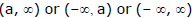] if it is differentiable at every number in the interval.

Derivability Over An Interval : f(x) is said to be derivable over an interval if it is derivable at each & every point of the interval.  f(x) is said to be derivable over the closed interval [a, b] if :

(i) for the points a and b, f '(a+) & f '(b -) exist &
(ii) for any point c such that a < c < b, f '(c+) & f'(c -) exist & are equal .

How Can a Function Fail to Be Differentiable ?

We see that the function y = |x| is not differentiable at 0 and Figure shows that its graph changes direction abruptly when x = 0. In general, if the graph of a function f has a "corner" or "kink" in it, then the graph of f has no tangent at this point and f is not differentiable there. [In trying to compute f '(a), we find that the left and right limits are different.]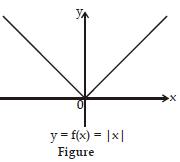There is another way for a function not to have a derivative. If f is discontinuous at a, then f is not differentiable at a. So at any discontinuity (for instance, a jump discontinuity), f fails to be differentiable.

A third possibility is that the curve has a vertical tangent line when at x = a,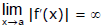This means that the tangent lines become steeper and steeper as x → a. Figure (a, b, c) illustrates the three posibilities that we have discussed.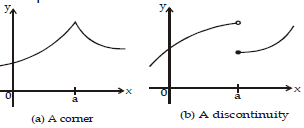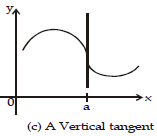Right hand & Left hand Derivatives By definition : f '(a) =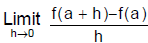(i) The right hand derivative of f ' at x = a denoted by f '+(a) is defined by :

f '+(a) =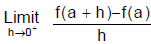, provided the limit exists & is finite.

(ii) The left hand derivative of f at x = a denoted by f '-(a) is defined by :

f ' -(a) =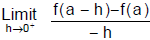, Provided the limit exists & is finite. We also write f '+(a) = f '(a+) & f '-(a) = f '(a-) .

f'(a) exists if and only if these one-sided derivatives exist and are equal.

Ex.20 If a function f is defined by f(x) =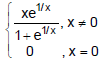show that f is continuous but not derivable at x = 0

Sol. We have f(0 + 0) =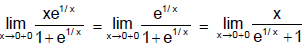= 0

f(0 - 0) =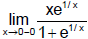= 0

Also f(0) = 0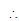f(0 + 0) = f(0 - 0) = f(0) ⇒ f is continuous at x = 0

Again f'(0 + 0) =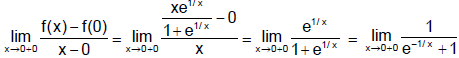= 1

f'(0 - 0) =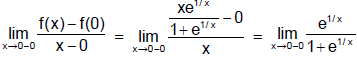= 0

Since f'(0 + 0)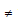f'(0 - 0), the derivative of f(x) at x = 0 does not exist.

Ex.21 A function f(x) is such that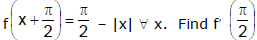, if it exists.

Sol. Given that =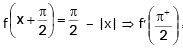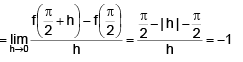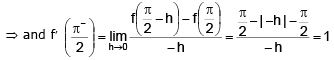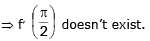Ex.22 Let f be differentiable at x = a and let f (a) ¹ 0. Evaluate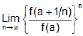.

Sol. l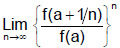(1 form)

l =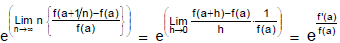(put n = 1/h)

Ex.23 Let f : R → R satisfying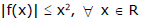then show f(x) is differentiable at x = 0.

Sol. Since,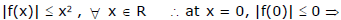f(0) = 0    ...(i)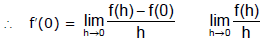....(ii) {f(0) = 0 from (i)}

Now,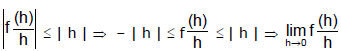→ 0 ...(iii) {using Cauchy-Squeeze theorem}from (ii) and (iii) , we get f'(0) = 0. i.e. f(x) is differentiable at x = 0.

F. Operation on Differentiable Functions

1. If f(x) & g(x) are derivable at x = a then the functions f(x) + g(x), f(x) - g(x), f(x). g(x) will also be derivable at x = a & if g (a)0 then the function f(x)/g(x) will also be derivable at x = a.

If f and g are differentiable functions, then prove that their product fg is differentiable.

Let a be a number in the domain of fg. By the definition of the product of two functions we have

(fg) (a) = f(a) g(a) (fg) (a + t) = f(a + t) g(a + t).

Hence (fg)' (a) =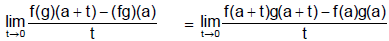The following algebraic manipulation will enable us to put the above fraction into a form in which we can see what the limit is:

f(a + t) g(a + t) - f(a) g(a) = f(a + t) g(a + t) - f(a) g (a + t) + f(a)g(a + t) - f(a) g(a)

= [f(a + t) - f(a)] g(a + t) + [g(a + t) - g(a)] f(a).

Thus (fg)' (a) =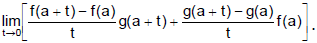.

The limit of a sum of products is the sum of the products of the limits. Moreover, f'(a) and g'(a) exist by hypothesis. Finally, since g is differentiable at a, it is continuous there ; and so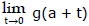= f(a). We conclude that

(fg)'(a) == f'(a)g(a) + g'(a)f(a) = (f'g + g'f) (a).

2. If f(x) is differentiable at x = a & g(x) is not differentiable at x = a , then the product function F(x) = f(x) . g(x) can still be differentiable at x = a e.g. f(x) = x  and g(x) =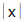.

3. If f(x) & g(x) both are not differentiable at x = a then the product function ;

F(x) = f(x) . g(x) can still be differentiable at x = a e.g. f(x) =& g(x) =4. If f(x) & g(x) both are non-deri. at x = a then the sum function F(x) = f(x) + g(x) may be a differentiable function . e.g. f(x) =& g(x) = -5. If f(x) is derivable at x = a ⇒ f '(x) is continuous at x = a.

e.g. f(x) =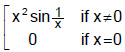G. Functional Equations

Ex.24 Let f(xy) = xf(y) + yf(x) for all x,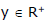and f(x) be differentiable in (0, ∞) then determine f(x).

Given f(xy)= xf(y) + yf(x)

Sol. Replacing x by 1 and y by x then we get x f(1) = 0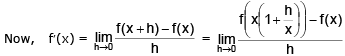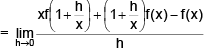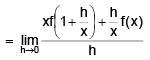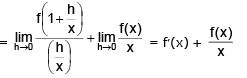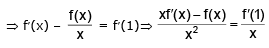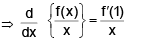On integrating w.r.t.x and taking limit 1 to x then f(x)/x - f(1)/1 = f'(1) (ln x – ln 1)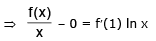∴  f(1) = 0) ∴  f(x) = f'(1) (x ln x)

Alternative Method :

Given f(xy) = xf(y) + yf(x)

Differentiating both sides w.r.t.x treating y as constant, f'(xy) . y = f(y) + yf'(x)

Putting y = x and x = 1, then

f'(xy). x = f(x) + xf'(x)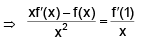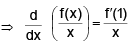Integrating both sides w.r.t.x taking limit 1 to x,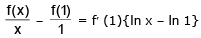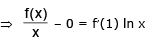Hence, f(x) =- f'(1)(x ln x).

Ex.25 If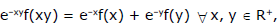and f'(1) = e, determine f(x).

Sol.

Given e–xy f(xy) = e–xf(x) + e–yf(y) ....(1)

Putting x = y = 1 in (1), we get f(1) = 0 ...(2)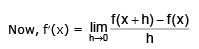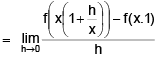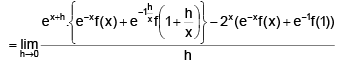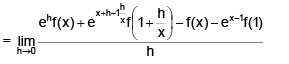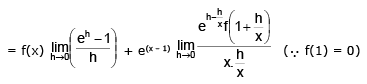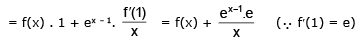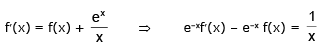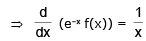On integrating we have e–xf(x) = ln x + c at x = 1, c = 0

∴  f(x) = ex ln x

Ex.26 Let f be a function such that f(x + f(y)) = f(f(x)) + f(y) x, y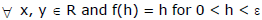where ε > 0, then determine f"(x) and f(x).

Sol. Given f(x + f(y)) = f(f(x) + f(y))  .....(1)

Put x = y = 0 in (1), then f(0 + f(0)) = f(f(0)) + f(0)  ⇒    f(f(0)) = f(f(0)) + f(0)

∴ f(0) = 0 ...(2)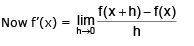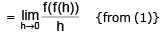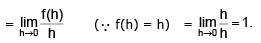Integrating both sides with limites 0 to x then f(x) = x   ∴ f'(x) = 1.

Offer running on EduRev: Apply code STAYHOME200 to get INR 200 off on our premium plan EduRev Infinity!

## Mathematics (Maths) Class 12

209 videos|222 docs|124 tests

,

,

,

,

,

,

,

,

,

,

,

,

,

,

,

,

,

,

,

,

,

;